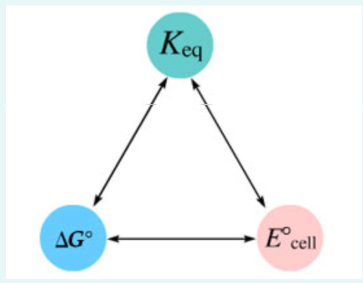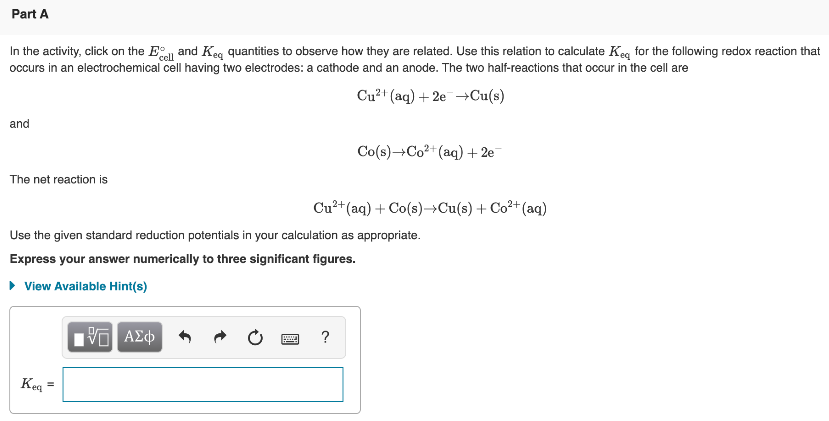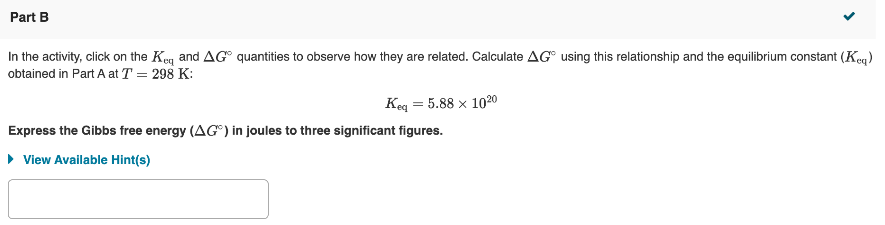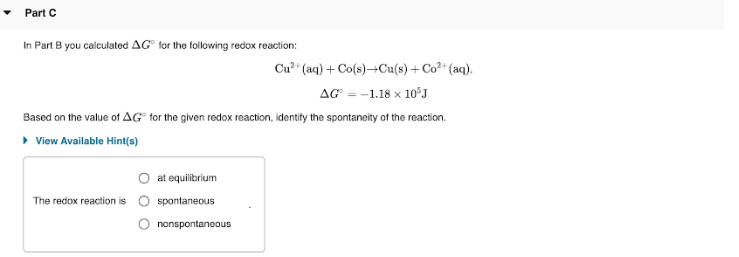# The following values may be useful when solving this tutorial. In the activity, click on the E° cell and Keq quantities to observe how they are related. Use this relation to calculate Keq for the following redox reaction that occurs in an electrochemical cell having two electrodes: a cathode and an anode. The two half-reactions that occur in the cell are Cu^2+(aq) + 2e^- → Cu(s) and Co(s) → Co^2+(aq) + 2e^- The net reaction is Cu^2+(aq) + Co(s) → Cu(s) + Co^2+(aq) Use the given standard reduction potentials in your calculations as appropriate. Express your answer numerically to three significant figures. In the activity, click on the Keq and ΔG° quantities to observe how they are related. Calculate ΔG° using this relationship and the equilibrium constant (Keq) obtained in Part A at = 298 K: Keq = 5.88 x 10^20 Express the Gibbs free energy ( ΔG°) in joules to three significant figures. In Part B you calculated ΔG° for the following redox reaction: Cu^2+(aq) + Co(s) → Cu(s) + Co^2+, ΔG° = -1.18 x 10^5J Based on the value of ΔG° for the given redox reaction, identify the spontaneity of the reaction. The redox reaction is a) at equilibrium b) spontaneous c) nonspontaneous